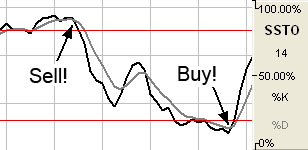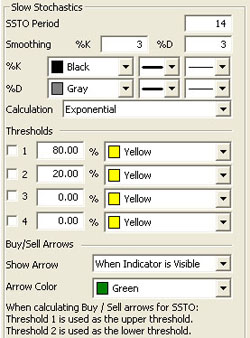# Slow Stochastics (SSTO)

The slower version of Stochastics is commonly believed to be a more reliable indicator. In this version of Stochastics, the more sensitive %K line is dropped. The original %D now becomes the slower line %K. The new %D is a 3-day moving average of the %K. This basically gives you a smoothed version of the original indictor. This modified counter trend indicator is less reactive but considered to be more accurate.

Slow Stochastics are interpreted the same as Fast Stochastics. Quite often the faster of the two indicators moves in and out of the overbought/oversold regions quickly.

### Calculation

The calculations for the slow stochastic are similar to the normal stochastic. The first step in computing the stochastic indicator is to determine the n period high and low. Suppose you specified twenty periods for the stochastic. Determine the highest high and lowest low during the last twenty trading intervals. It determines the trading range for that time period. The trading range changes on a continuous basis.

The calculations for the %K is here:

%Kt = ( (Closet - Lown) / (Highn - Lown) ) x 100

%Kt: The value for the first %K for the current time period.
Closet: The closing price for the current period.
Lown: The lowest low during the n periods.
Highn: The highest high during the n time periods.
n: The value you specify.

Once you obtain the %K value, you start computing the %D value, which is an accumulative moving average. Since the %D is a moving average of a moving average, it requires several trading intervals before the values are calculated properly. If you specify a 20 period stochastic, the software system requires 26 trading intervals before it can calculate valid %K and %D values. The formula for the %D is here:

%DT = ( (%DT-1 x 2) + %Kt) / 3

%DT: The value for %D in the current period.
%DT-1: The value for %D in the previous time period.
%Kt: The value for %K in the current period.

The values 2 and 3 are constants. You specify the constants and the length of the time period to examine for the trading range.

Once the %K and %D values for the normal stochastic are derived, the slow stochastic can be computed. The formula for the slow stochastic is here:

%KSLOW = %DNORMAL
%DSLOWt = ( ( %D SLOWt-1 x 2 ) + %K SLOWt-1 ) ) / 3

%KSLOW: The %D for the normal stochastic.
%DSLOWt: Slow %D value for the current period.
%DSLOWt-1: The slow %D for the previous period.
%KSLOWt-1: The slow %K for the previous period.

The values 2 and 3 are the smoothing constants. You may select different values.

A buy signal occurs when the %K line crosses from below %D to above %D and both lines are less than the lower threshold. A sell signal occurs when the %K line crosses from above the %D line to below the %D line and both lines are greater than the upper threshold.### Preferences

Right-click on the SSTO button in your Indicator toolbar and select SSTO Settings. The Preferences Tab will open in the Control Panel and the SSTO preferences will be displayed. (Once you click on the chart, the Preference tab will go back to chart settings.)

Restore Settings: TNT Default will change your settings back to the original software settings. My Default will change current settings to your personalized default settings. Apply To All Charts will apply your selected settings on all open charts. Save As My Default will save your current personal settings.SSTO Period: The number of periods used to determine the highest high and lowest low. Default is 14.

Smoothing: The number of periods used to determine the moving average for the %K and %D values.

%K/%D: Choose the color, line style, and line thickness of your %K and %D lines.

Calculation: Choose between Exponential, Simple, and Wilder's Smoothing calculations.

View up to four Thresholds at values and colors of your choice. When calculating buy/sell signals, Threshold 1 is used as the upper threshold and Threshold 2 is used as the lower threshold (default values set at 80 and 20).

Choose when you want Buy/Sell Arrows to show and what color.